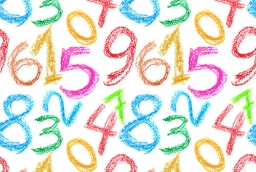# Probability 17013

What is the probability that a randomly written two-digit number from number 20 to number 99 will be divisible by 11, the power of number 3, or a prime number?

p =  0.3375

### Step-by-step explanation:Did you find an error or inaccuracy? Feel free to write us. Thank you!

Tips for related online calculators
Would you like to compute the count of combinations?